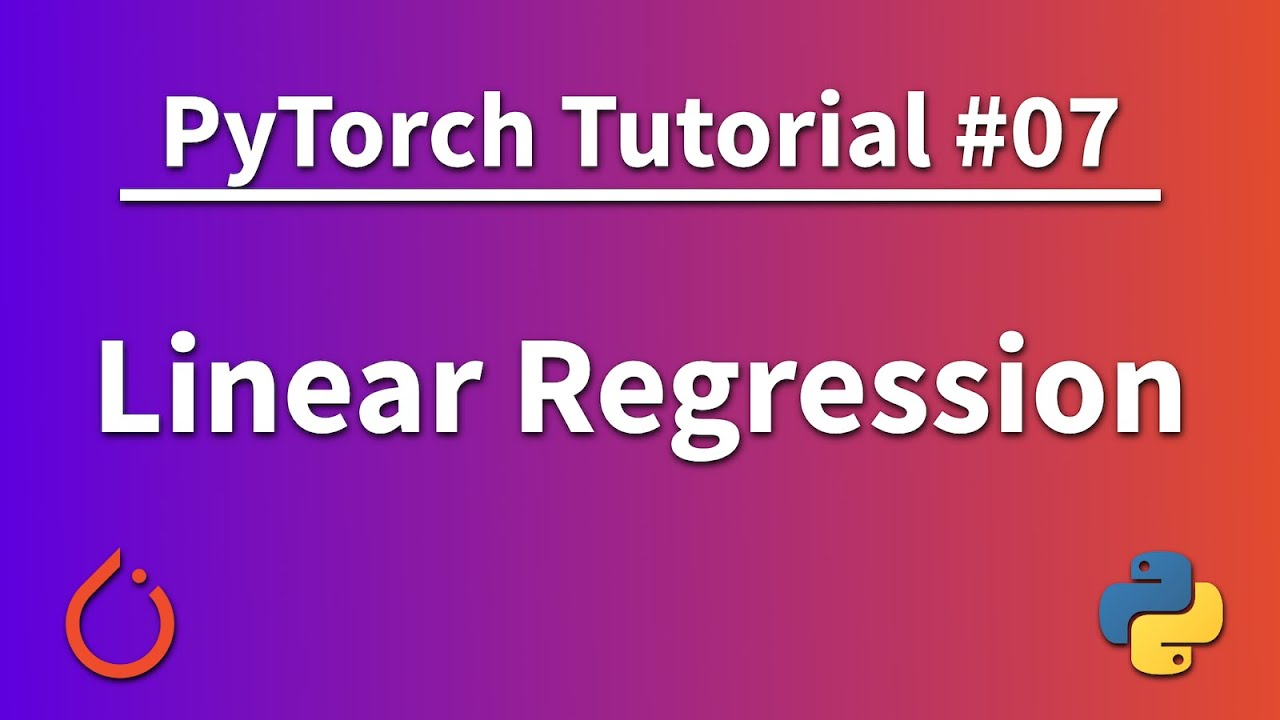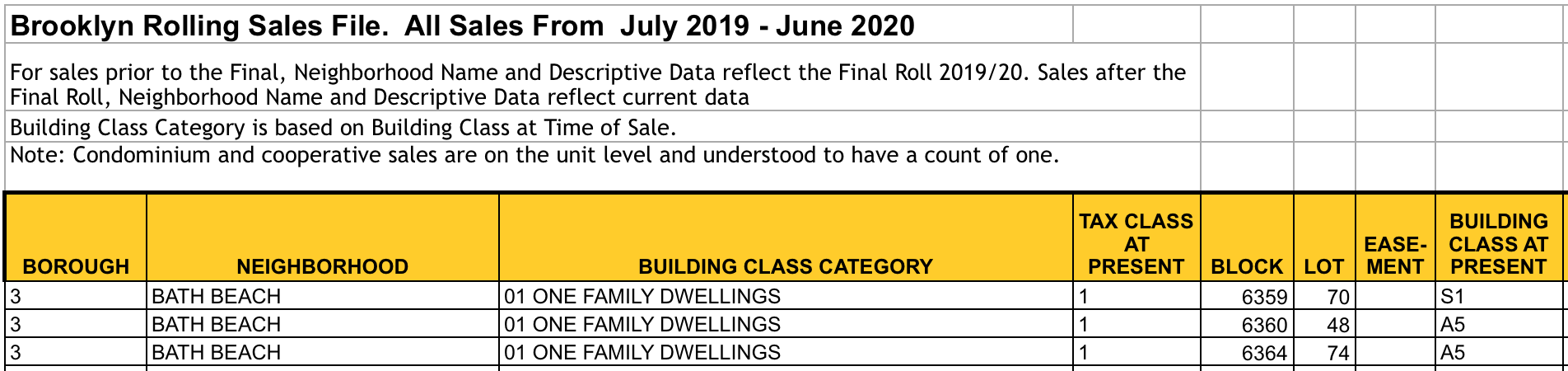1577678520

# PyTorch Tutorial - Linear Regression

Learn all the basics you need to get started with this deep learning framework! In this part we implement a logistic regression algorithm and apply all the concepts that we have learned so far:

• Training Pipeline in PyTorch
• Model Design
• Loss and Optimizer
• Automatic Training steps with forward pass, backward pass, and weight updates

Official website:
https://pytorch.org/

Code for this tutorial series:
https://github.com/python-engineer/pytorchTutorial

#python #pytorch #deep-learning #programming #developer

## Buddha Community1594271340

## A Deep Dive into Linear Regression

Let’s begin our journey with the truth — machines never learn. What a typical machine learning algorithm does is find a mathematical equation that, when applied to a given set of training data, produces a prediction that is very close to the actual output.

Why is this not learning? Because if you change the training data or environment even slightly, the algorithm will go haywire! Not how learning works in humans. If you learned to play a video game by looking straight at the screen, you would still be a good player if the screen is slightly tilted by someone, which would not be the case in ML algorithms.

However, most of the algorithms are so complex and intimidating that it gives our mere human intelligence the feel of actual learning, effectively hiding the underlying math within. There goes a dictum that if you can implement the algorithm, you know the algorithm. This saying is lost in the dense jungle of libraries and inbuilt modules which programming languages provide, reducing us to regular programmers calling an API and strengthening further this notion of a black box. Our quest will be to unravel the mysteries of this so-called ‘black box’ which magically produces accurate predictions, detects objects, diagnoses diseases and claims to surpass human intelligence one day.

We will start with one of the not-so-complex and easy to visualize algorithm in the ML paradigm — Linear Regression. The article is divided into the following sections:

1. Need for Linear Regression

2. Visualizing Linear Regression

3. Deriving the formula for weight matrix W

4. Using the formula and performing linear regression on a real world data set

Note: Knowledge on Linear Algebra, a little bit of Calculus and Matrices are a prerequisite to understanding this article

Also, a basic understanding of python, NumPy, and Matplotlib are a must.

## 1) Need for Linear regression

Regression means predicting a real valued number from a given set of input variables. Eg. Predicting temperature based on month of the year, humidity, altitude above sea level, etc. Linear Regression would therefore mean predicting a real valued number that follows a linear trend. Linear regression is the first line of attack to discover correlations in our data.

Now, the first thing that comes to our mind when we hear the word linear is, a line.

Yes! In linear regression, we try to fit a line that best generalizes all the data points in the data set. By generalizing, we mean we try to fit a line that passes very close to all the data points.

But how do we ensure that this happens? To understand this, let’s visualize a 1-D Linear Regression. This is also called as Simple Linear Regression

#calculus #machine-learning #linear-regression-math #linear-regression #linear-regression-python #python1592023980

## 5 Regression algorithms: Explanation & Implementation in Python

Take your current understanding and skills on machine learning algorithms to the next level with this article. What is regression analysis in simple words? How is it applied in practice for real-world problems? And what is the possible snippet of codes in Python you can use for implementation regression algorithms for various objectives? Let’s forget about boring learning stuff and talk about science and the way it works.

#linear-regression-python #linear-regression #multivariate-regression #regression #python-programming1598352300

## Regression: Linear Regression

Machine learning algorithms are not your regular algorithms that we may be used to because they are often described by a combination of some complex statistics and mathematics. Since it is very important to understand the background of any algorithm you want to implement, this could pose a challenge to people with a non-mathematical background as the maths can sap your motivation by slowing you down.In this article, we would be discussing linear and logistic regression and some regression techniques assuming we all have heard or even learnt about the Linear model in Mathematics class at high school. Hopefully, at the end of the article, the concept would be clearer.

**Regression Analysis **is a statistical process for estimating the relationships between the dependent variables (say Y) and one or more independent variables or predictors (X). It explains the changes in the dependent variables with respect to changes in select predictors. Some major uses for regression analysis are in determining the strength of predictors, forecasting an effect, and trend forecasting. It finds the significant relationship between variables and the impact of predictors on dependent variables. In regression, we fit a curve/line (regression/best fit line) to the data points, such that the differences between the distances of data points from the curve/line are minimized.

#regression #machine-learning #beginner #logistic-regression #linear-regression #deep learning1596728880

## Tutorial: Getting Started with R and RStudio

In this tutorial we’ll learn how to begin programming with R using RStudio. We’ll install R, and RStudio RStudio, an extremely popular development environment for R. We’ll learn the key RStudio features in order to start programming in R on our own.

If you already know how to use RStudio and want to learn some tips, tricks, and shortcuts, check out this Dataquest blog post.

#data science tutorials #beginner #r tutorial #r tutorials #rstats #tutorial #tutorials1596513720

## 1. Characteristics of Clean Data and Messy Data

What exactly is clean data? Clean data is accurate, complete, and in a format that is ready to analyze. Characteristics of clean data include data that are:

• Free of duplicate rows/values
• Error-free (e.g. free of misspellings)
• Relevant (e.g. free of special characters)
• The appropriate data type for analysis
• Free of outliers (or only contain outliers have been identified/understood), and
• Follows a “tidy data” structure

Common symptoms of messy data include data that contain:

• Special characters (e.g. commas in numeric values)
• Numeric values stored as text/character data types
• Duplicate rows
• Misspellings
• Inaccuracies
• White space
• Missing data
• Zeros instead of null values

## 2. Motivation

In this blog post, we will work with five property-sales datasets that are publicly available on the New York City Department of Finance Rolling Sales Data website. We encourage you to download the datasets and follow along! Each file contains one year of real estate sales data for one of New York City’s five boroughs. We will work with the following Microsoft Excel files:

• rollingsales_bronx.xls
• rollingsales_brooklyn.xls
• rollingsales_manhattan.xls
• rollingsales_queens.xls
• rollingsales_statenisland.xls

As we work through this blog post, imagine that you are helping a friend launch their home-inspection business in New York City. You offer to help them by analyzing the data to better understand the real-estate market. But you realize that before you can analyze the data in R, you will need to diagnose and clean it first. And before you can diagnose the data, you will need to load it into R!

Benefits of using tidyverse tools are often evident in the data-loading process. In many cases, the tidyverse package `readxl` will clean some data for you as Microsoft Excel data is loaded into R. If you are working with CSV data, the tidyverse `readr` package function `read_csv()` is the function to use (we’ll cover that later).

Let’s look at an example. Here’s how the Excel file for the Brooklyn borough looks:The Brooklyn Excel file

Now let’s load the Brooklyn dataset into R from an Excel file. We’ll use the `readxl`package. We specify the function argument `skip = 4` because the row that we want to use as the header (i.e. column names) is actually row 5. We can ignore the first four rows entirely and load the data into R beginning at row 5. Here’s the code:

``````library(readxl) # Load Excel files
brooklyn <- read_excel("rollingsales_brooklyn.xls", skip = 4)
``````

Note we saved this dataset with the variable name `brooklyn` for future use.

## 4. View the Data with tidyr::glimpse()

The tidyverse offers a user-friendly way to view this data with the `glimpse()` function that is part of the `tibble` package. To use this package, we will need to load it for use in our current session. But rather than loading this package alone, we can load many of the tidyverse packages at one time. If you do not have the tidyverse collection of packages, install it on your machine using the following command in your R or R Studio session:

``````install.packages("tidyverse")
``````

Once the package is installed, load it to memory:

``````library(tidyverse)
``````

Now that `tidyverse` is loaded into memory, take a “glimpse” of the Brooklyn dataset:

``````glimpse(brooklyn)
## Observations: 20,185
## Variables: 21
## \$ BOROUGH <chr> "3", "3", "3", "3", "3", "3", "…
## \$ NEIGHBORHOOD <chr> "BATH BEACH", "BATH BEACH", "BA…
## \$ `BUILDING CLASS CATEGORY` <chr> "01 ONE FAMILY DWELLINGS", "01 …
## \$ `TAX CLASS AT PRESENT` <chr> "1", "1", "1", "1", "1", "1", "…
## \$ BLOCK <dbl> 6359, 6360, 6364, 6367, 6371, 6…
## \$ LOT <dbl> 70, 48, 74, 24, 19, 32, 65, 20,…
## \$ `EASE-MENT` <lgl> NA, NA, NA, NA, NA, NA, NA, NA,…
## \$ `BUILDING CLASS AT PRESENT` <chr> "S1", "A5", "A5", "A9", "A9", "…
## \$ ADDRESS <chr> "8684 15TH AVENUE", "14 BAY 10T…
## \$ `APARTMENT NUMBER` <chr> NA, NA, NA, NA, NA, NA, NA, NA,…
## \$ `ZIP CODE` <dbl> 11228, 11228, 11214, 11214, 112…
## \$ `RESIDENTIAL UNITS` <dbl> 1, 1, 1, 1, 1, 1, 1, 1, 2, 1, 1…
## \$ `COMMERCIAL UNITS` <dbl> 1, 0, 0, 0, 0, 0, 0, 0, 0, 0, 0…
## \$ `TOTAL UNITS` <dbl> 2, 1, 1, 1, 1, 1, 1, 1, 2, 1, 1…
## \$ `LAND SQUARE FEET` <dbl> 1933, 2513, 2492, 1571, 2320, 3…
## \$ `GROSS SQUARE FEET` <dbl> 4080, 1428, 972, 1456, 1566, 22…
## \$ `YEAR BUILT` <dbl> 1930, 1930, 1950, 1935, 1930, 1…
## \$ `TAX CLASS AT TIME OF SALE` <chr> "1", "1", "1", "1", "1", "1", "…
## \$ `BUILDING CLASS AT TIME OF SALE` <chr> "S1", "A5", "A5", "A9", "A9", "…
## \$ `SALE PRICE` <dbl> 1300000, 849000, 0, 830000, 0, …
## \$ `SALE DATE` <dttm> 2020-04-28, 2020-03-18, 2019-0…
``````

The `glimpse()` function provides a user-friendly way to view the column names and data types for all columns, or variables, in the data frame. With this function, we are also able to view the first few observations in the data frame. This data frame has 20,185 observations, or property sales records. And there are 21 variables, or columns.

#data science tutorials #beginner #r #r tutorial #r tutorials #rstats #tidyverse #tutorial #tutorials